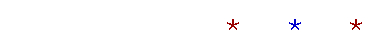Topics in

P R E C A L C U L U S

23

# FACTORIALS

BY THE SYMBOL n! ("n factorial") we mean the product of consecutive numbers 1 through n.

 n! = 1· 2· 3·  ·  · (n − 2)(n − 1)n = n(n − 1)(n − 2)·  ·  · 3· 2· 1

The order of the factors does not matter, whether backwards or forwards.

0! is defined as 1.

0! = 1.

(The usefulness of this definition will become clear as we continue.)

Example.   6! = 1· 2· 3· 4· 5· 6 = 720

A convenient way to calculate this is to wait to multiply 2· 5 = 10.  Then

3· 4· 6 = 12· 6 = 72

-- times 10 is 720.

Calculations with factorials are based on this fact:

Any factorial less than n! is a factor of n!.

Example 1.   6! is a factor of 10!.  For,

 10! = 1· 2· 3· 4· 5· 6· 7· 8· 9· 10 = 6!· 7· 8· 9· 10
 Example 2.   Evaluate 8!5! .
 8!5! = 1· 2· 3· 4· 5· 6· 7· 8       1· 2· 3· 4· 5 = 6· 7· 8 = 6· 56 = 336
 Example 3.   Evaluate 8!  6! 2! .

Solution.  6! is a factor of 8!, so it will cancel  leaving 7· 8 in the

 numerator.  We will have 7· 81· 2 = 28.

Problem 1.   What factorial is each of these?  (Consider what each symbol means.)

a)  3!· 4  = 4!  For, 3!· 4 = 1· 2· 3· 4 = 4!.

b)  6!· 7· 8  = 8!

c)  (n − 1)! n  = n!

d)  (nk − 1)! (nk) = (nk)!

 Problem 2.   Evaluate 10! 7! .
 10! 7! = 7!· 8· 9· 10      7! = 8· 9· 10 = 720
 Problem 3.   Evaluate 12!   10! 2! .
 12!   10! 2! = 10!· 11· 12   10! 2! = 11· 12  1· 2 = 11· 6 = 66
 Example 4.   Show: 1     (n − 1)! = n n!

Solution.  If we multiply both terms on the left by n, then

 1     (n − 1)! = n      (n − 1)! n

Now, (n − 1)! is the product up to the number just before n.  Therefore, (n − 1)! times n itself  is n!.

 1     (n − 1)! = n      (n − 1)! n = n n!

Example 5.   Show:  n! k  +  n! (nk + 1) = (n + 1)!

Solution.   On the left-hand side, n! is a common factor:

 n! k  +  n! (n − k + 1) = n! (k + n − k + 1) = n! (n + 1),   on canceling the k's, = (n + 1)!

(n + 1) is the number after n.

Problem 4.   Write out the steps that show how to transform the left-hand side into the right-hand side.  Again, consider what each symbol means.

 a) 13! = 44!

Multiply both terms on the left by 4:

 1 3! = 4  3!· 4 = 44!
 b) 1     (k − 1)! = k k!

Multiply both terms on the left by k:

 1    (k − 1)! = k      (k − 1)!· k = k k!
 c) 12 12! = 1 11!

Since 12! = 11!· 12, divide both terms on the left by 12:

 12 12! = 12   11!· 12 = 1 11!
 d) n − k  (n − k)! = 1        (n − k − 1)!

Since (nk)! = (nk − 1)!· (nk), divide both terms on the left by (n − k):

 n − k  (n − k)! = n − k           (n − k − 1)!· (n − k) = 1        (n − k − 1)!

e)   5!· 4  +  5!· 2 = 6!

5! is a common factor:

5!· 4  +  5!· 2 = 5!(4 + 2) = 5!· 6 = 6!Next Topic:  Permutations and combinations

Please make a donation to keep TheMathPage online.
Even \$1 will help.